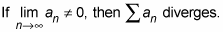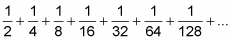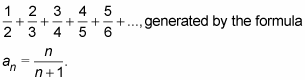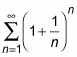##### Trigonometry Workbook For DummiesIf the individual terms of a series (in other words, the terms of the series’ underlying sequence) do not converge to zero, then the series must diverge. This is the nth term test for divergence. This is usually a very easy test to use.

The nth Term Test:(You probably figured out that with this naked summation symbol, n runs from 1 to infinity.)

If you think about it, this is just common sense. When a series converges, the sum of all the terms is honing in on a certain number. The only way this can happen is when the numbers being added at the far “end” of the series are getting infinitesimally small — like in the series:Imagine, instead, that the terms of a series are converging, say, to 1, like in the seriesIn that case, when you add up the terms, you keep adding on numbers extremely close to 1 over and over and over forever — and this must add up to infinity. So, in order for a series to converge, the terms of the series must converge to zero. But make sure you understand what this nth term test does not say.

When the terms of a series converge to zero, that does not guarantee that the series converges. In hifalutin logicianese — the fact that the terms of a series converge to zero is a necessary but not sufficient condition for concluding that the series converges to a finite sum.

Because this test is usually very easy to apply, it should be one of the first things you check when trying to determine whether a series converges or diverges. For example, if you’re asked to determine whetherconverges or diverges, note that every term of this series is a number greater than 1 being raised to a positive power. This always results in a number greater than 1, and thus, the terms of this series do not converge to zero, and the series must therefore diverge.

The nth term test not only works for ordinary positive series, but it also works for series with positive and negative terms.